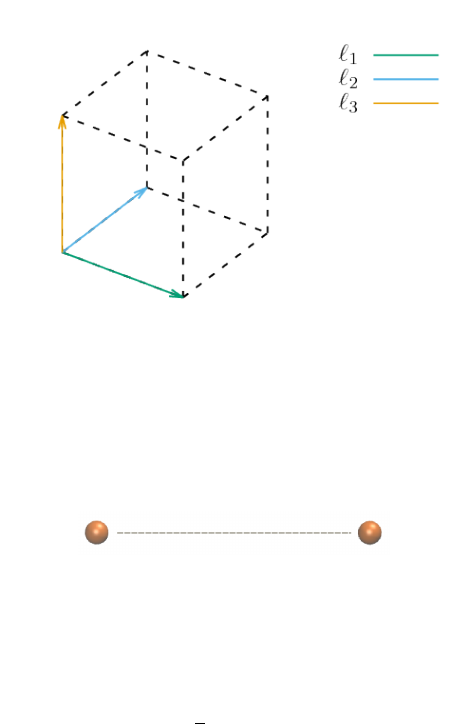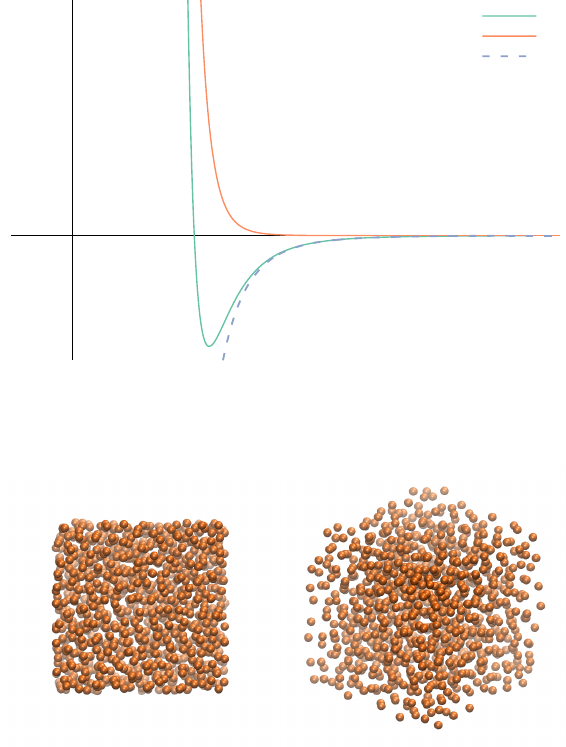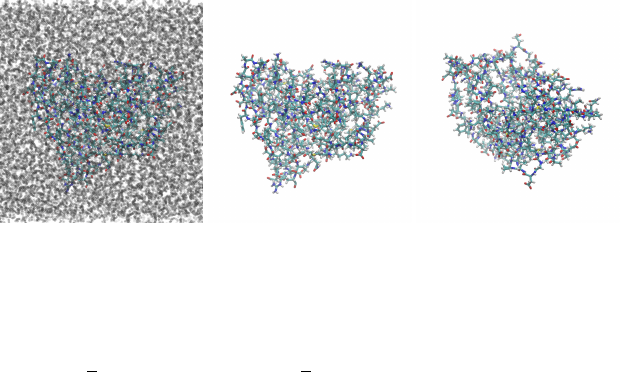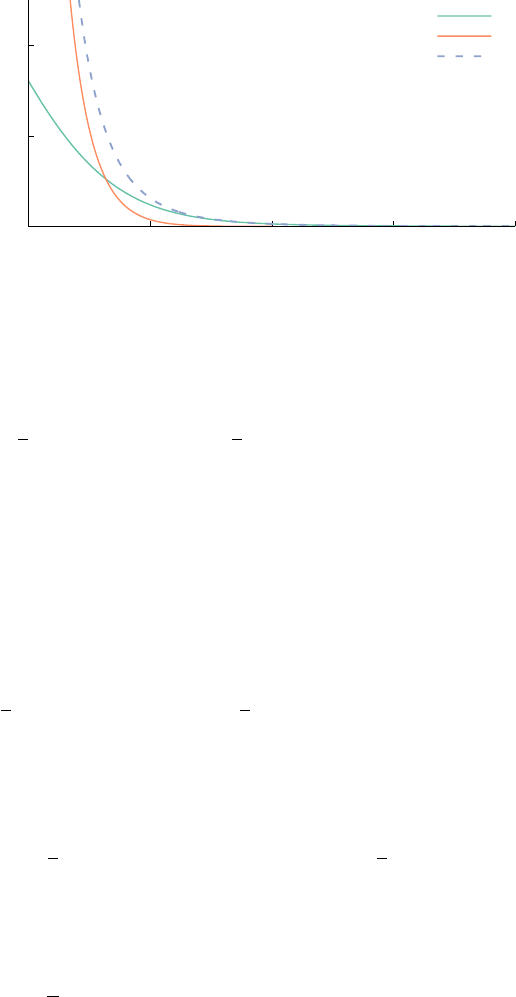Tutorial on the smooth particle-mesh Ewald algo-
rithm
These notes explain the smooth particle-mesh Ewald algorithm (SPME)
in detail. The accompanying library libpme6 is a fully-featured imple-
mentation of SPME that can be used for experimentation and reference.
This content can also be found in PDF format here.
Introduction
Motivation and overview of the SPME algorithm
Consider two atoms, labeled 1 and 2, contained in a parallelepiped
with sides determined by the (positively oriented) vectors `
1
, `
2
, `
3
R
3
(see Figure ). Then the matrix L = [`
1
, `
2
, `
3
] R
3×3
is such
Figure 1: Simulation box spanned by
three vectors.
that V = det L > 0. Now suppose that atom i is at a position r
i
=
L x
i
where x
i
[0, 1)
3
for i = 1, 2 and that r
ij
= kr
i
r
j
k (see
Figure ). Between these two atoms there is a force that depends on
Figure 2: Two atoms at a distance r
ij
.
their distance and whose corresponding potential energy, U
ij
=
U
ij
(r
ij
), is shown in Figure . If instead of just two atoms we had a
total of N > 2 atoms (see Figure ), then the total potential energy
would be equal to
H =
1
2
N
i=1
N
j=1
i6=j
U
ij
(r
ij
). (1)
By solving Newton’s second law
1
we would be able to simulate the
1
Force equals mass times acceleration.tutorial on spme 2
Energy
Distance (r)
U(r) = r
12
r
6
U
0
(r) = r
12
U
1
(r) = r
6
Figure 3: Decomposition of the Lennard
Jones 12-6 potential into repulsive
(powers of 12) and dispersive (powers
of 6) contributions.
Figure 4: Argon gas (N = 10
3
atoms).
molecular system of N atoms that interact according to the (pairwise)
forces given by F = U. In practice, whatever value of N that can
be tractable with current computers is still far away from the more
realistic ﬁgure of N
A
= 6.022 × 10
23
would capture the real physics of the system.
One trick to somehow get closer to N
A
is to use a tractable value
of N and impose periodic boundary conditions (PBCs). This means
that we now have an inﬁnite lattice of simulation boxes with identical
copies of the particles in each box. By computing the interactions
between the atoms in the original box and their copies (only the
copies in the neighboring simulation boxes are typically taken into
account —this is called the minimum image convention—), we can
make our simulations more realistic.tutorial on spme 3
Figure 5: The protein DHFR solvated
in water (left) and two views (center,
right) without solvent. This system has
N = 23536 atoms, so N
2
10
8
and
N log
2
N 10
5
.
The total potential energy (1) then becomes
H =
1
2
N
i=1
N
j=1
i6=j
U
ij
(kr
i
r
j
k) +
1
2
nZ
3
n6=0
N
i=1
N
j=1
U
ij
(kr
i
r
j
+ Lnk).
The naive approach to PBCs requires O(N
2
) calculations and is
unfeasible, hence the interest of ﬁnding efﬁcient ways of carrying
2
2
P. P. Ewald. Die Berechnung optischer
und elektrostatischer Gitterpotentiale.
Annalen der Physik, 369(3):253287, 1921
Harmonic analysis to reduce the complexity to O(N
3/2
) operations,
which is still impractical if we want to solve the equations of mo-
tion of a large system. The Particle-Mesh Ewald (PME) method
3
3
T. Darden, D. York, and L. Pedersen.
Particle mesh Ewald: An N log(N)
method for Ewald sums in large sys-
tems. The Journal of Chemical Physics,
98(12):10089, 1993
uses the Fast Fourier Transform to bring the complexity down to
O(N log N), and this algorithm has been a staple of Molecular Simu-
lation since its appearance. The Smooth Particle-Mesh Ewald (SPME)
improves on PME by giving a sufﬁciently smooth energy function
whose derivative can be obtained analytically, which results in more
realistic simulations due to improved energy conservation.
Some of the people involved in the development of the SPME
algorithm appear in Figure .
Smooth Particle-Mesh Ewald
We derive the formulas for Ewald summation following
4
and then
4
D. E. Williams. Accelerated conver-
gence treatment of R
n
lattice sums. In
Uri Shmueli, editor, International Tables
for Crystallography, volume B, pages
2006
we introduce the smooth Particle-Mesh Ewald method from
5
.
5
U. Essmann, L. Perera, M. L.
Berkowitz, T. Darden, H. Lee, and
L. G. Pedersen. A smooth particle mesh
Ewald method. The Journal of Chemical
Physics, 103(19):8577, 1995
Decomposition of the potential energy function
Ewald summation can be applied whenever the pairwise potential
energy U = U(r) is proportional to r
n
for some n N (a concrete
instance of U appears in Figure ). In that case, we can write U as a
sum of the form
U(r) = U
0
(r) + U
1
(r), (2)tutorial on spme 4
(a) Paul Peter Ewald
(1888-1985).
(b) Tom Darden and
Lee Pedersen.
(c) Mike Crowley.
Figure 6: Some of the people behind
SPME. Pictures reproduced from
http://journals.iucr.org/a/issues/
2012/01/00/wx0006/ and http://www.
psc.edu/science/Darden/.
where the short-range term, U
0
, decays fast as r goes to inﬁnity (so
that it can be truncated) and the long-range term, U
1
out and smooth, so that its Fourier transform is concentrated (and
can also be truncated).
There are many ways of splitting the potential energy but
6
estab-
6
B. R. A. Nijboer and F. W. De Wette.
On the calculation of lattice sums.
Physica, 23(1-5):309321, 1957
lishes the optimality for a class of functions involving the (incom-
plete) Gamma function. If we deﬁne
ϕ
n
(r) = 1
Γ(n/2, α
2
π r
2
)
Γ(n/2)
,
where α
2
is a parameter to be discussed later, then we clearly have
1
r
n
=
1
r
n
(
ϕ
n
(r) + (1 ϕ
n
(r))
)
.
We are going to concern ourselves with the case n = 6 (see Figure ),
and to deﬁne ψ( r) = r
6
ϕ
6
(r).
The free parameter α is determined by numerically solving the
equation
r
6
(
1 ϕ
6
(r
cutoff
; α)
)
= ε
with prescribed values of r
cutoff
and ε (the function 1 ϕ
n
must be
decreasing for r > r
cutoff
). This makes the short-range part of the
computation involving effectively vanish for r > r
cutoff
.tutorial on spme 5
0
0.05
0.1
1 2 3 4 5
Energy
r
r
6
ϕ
6
(r)
r
6
(1 ϕ
6
(r))
r
6
Figure 7: Decomposition of the function
r
6
into short-range and long-range
terms.
Recall that in the case of N > 2 particles, we have
H =
1
2
N
i=1
N
j=1
i6=j
U
ij
(kr
i
r
j
k) +
1
2
nZ
3
n6=0
N
i=1
N
j=1
U
ij
(kr
i
r
j
+ Lnk).
where r
ij
is the distance between atoms i and j. We can split the
pairwise potential U
ij
above as in (2).
Ewald summation
The summation of the long-range terms (with b
i
> 0),
U
1
=
1
2
N
i=1
N
j=1
i6=j
b
i
b
j
ψ(kr
i
r
j
k) +
1
2
nZ
3
n6=0
N
i=1
N
j=1
b
i
b
j
ψ(kr
i
r
j
+ Lnk)
(3)
can be rearranged as
U
1
=
1
2
nZ
3
N
i=1
N
j=1
b
i
b
j
ψ(kr
i
r
j
+ Lnk)
1
2
N
i=1
b
2
i
ψ(0), (4)
where ψ(0) = lim
r0
+
ψ(r).
Lemma 1 (Poisson’s summation formula). If D(r) =
nZ
3
δ(r Ln),
then
b
D(h) =
1
V
dZ
3
δ(h L
T
d).
Lemma 2. Consider the distributions ρ(r) =
N
i=1
b
i
δ(r r
i
), η(r) =
ρ(r), and D(r) =
nZ
3
δ(r Ln). The following identity holds:
nZ
3
N
i=1
N
j=1
b
i
b
j
ψ(kr
i
r
j
+ Lnk) =
Z
ψ(r)
(
ρ ? η ? D
)
(r) dr,
where r = krk.tutorial on spme 6
4 2 0 2 4
-4 -2 0 2 4
D
b
D
Figure 8: Example of Poisson’s summa-
tion formula.
Proof. First,
ρ ? η (r) =
Z
ρ(s) η(r s) ds
=
Z
ρ(s) ρ(s r) ds
=
N
i=1
N
j=1
b
i
b
j
Z
δ(s r
i
) δ(r s r
j
) ds
=
N
i=1
N
j=1
b
i
b
j
δ(r (r
i
r
j
)).
By the associative property of the convolution, we have
ρ ? η ? D =
(
ρ ? η
)
? D,
so
ρ ? η ? D (r) =
Z
ρ ? η (s) D(s r) ds